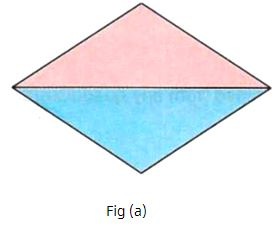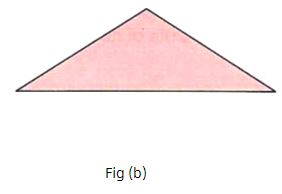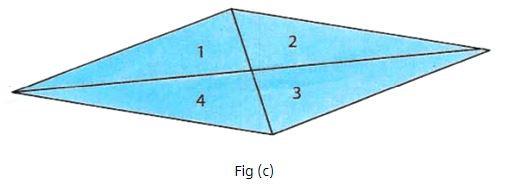Courses

# Procedure - To explore Similarities & Differences in properties of the Quadrilaterals, Class 9 Math Class 9 Notes | EduRev

## Mathematics (Maths) Class 9

Created by: Indu Gupta

## Class 9 : Procedure - To explore Similarities & Differences in properties of the Quadrilaterals, Class 9 Math Class 9 Notes | EduRev

The document Procedure - To explore Similarities & Differences in properties of the Quadrilaterals, Class 9 Math Class 9 Notes | EduRev is a part of the Class 9 Course Mathematics (Maths) Class 9.
All you need of Class 9 at this link: Class 9

Materials required:

Chart papers, pencil, compass, scale and a pair of scissors.

Property 1:

A diagonal of a parallelogram divides it into two congruent triangles.

As performed in real lab:

Procedure:

1. Make a parallelogram on a chart paper and cut it.

2. Draw diagonal of the parallelogram [Fig(a)].

3. Cut along the diagonal and obtain two triangles.

4. Superimpose one triangle onto the other [Fig(b)]As performed in the simulator:

1. Select three points A, B and C anywhere on the workbench  to draw a parallelogram.

2. Now, click on any of the vertices to draw a diagonal. The diagonal divides the parallelogram into two triangles - a green colored triangle and blue colored triangle.

3. Now, rotate the green triangle appropriately and drag to overlap blue triangle. Use rotation controls (r+ and r-) in "Tools" to rotate green triangle.

4. See the observations and inference.

Observations:

Two triangles are congruent to each other.

Property 2:

Diagonals of a parallelogram bisect each other.

As performed in real lab:

Procedure:

1. Draw the parallelogram and its both diagonals.

2. Cut the four triangles formed. Name them 1, 2, 3 and 4 [Fig(c)].

3. Observe that triangle 2 is congruent to triangle 4 and triangle 1 is congruent to triangle 3 by superimposing them on each other.

As performed in the simulator:

1. Select three points A, B and C anywhere on the workbench to draw a parallelogram.

2. Now, click on any two adjacent vertices to draw two diagonals. The diagonals divide the parallelogram into 4 triangles (two sets of opposite triangles)

3. Click on "Next" to color the four triangles. One set of opposite triangle is colored blue and green, while other is colored brown and violet.

4. Now, Rotate and Drag blue triangle over green triangle and brown triangle over violet triangle. Use rotation controls (r+ and r-) in "Tools" to rotate blue and brown triangles.

5. See observations and inference.

Observations:

• Base of triangle 2 = Base of triangle 4

• Base of triangle 1 = Base of triangle 3

• Thus the diagonals bisect each other.

## Mathematics (Maths) Class 9

192 videos|230 docs|82 tests

,

,

,

,

,

,

,

,

,

,

,

,

,

,

,

,

,

,

,

,

,

,

,

,

;